# ML Aggarwal Solutions Class 10 Maths Chapter 22 Probability

1. A bag contains a red ball, a blue ball and a yellow ball, all the balls being of the same size. Anjali takes out a ball from the bag without looking into it. What is the probability that she takes out
(i) yellow ball ?
(ii) red ball ?
(iii) blue ball ?
Solution:
Anjali takes out a ball from the bag without looking into it. So, it is equally likely that she takes out any one of them.
Let Y be the event ‘the ball taken out is yellow’,
B be the event ‘the ball taken out is blue’, and R be the event ‘the ball taken out is red’.
Now, we have given that
Number of possible outcomes = 3.
(i) The number of outcomes favourable to the event Y = 1.
∴probability of getting yellow ball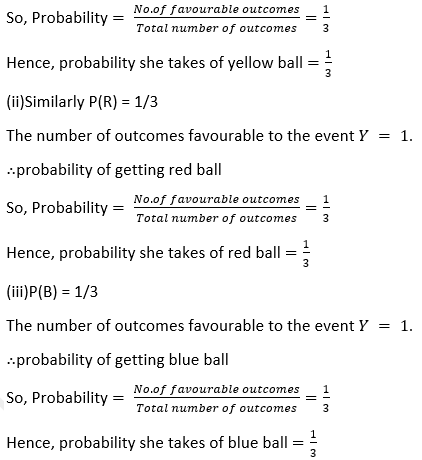2. A box contains 600 screws, one-tenth are rusted. One screw is taken out at random from this box. Find the probability that it is a good screw.
Solution:
We have given that,
Total number of screws = 600
Number of possible outcomes = 600
Number of rusted screws = one tenth of 600
= (1/10)×600 = 60
So, rusted screw =60
Number of remaining good screws = 600-60 = 540
Number of favourable outcomes = 540
Now,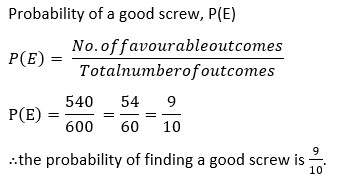3. In a lottery, there are 5 prized tickets and 995 blank tickets. A person buys a lottery ticket. Find the probability of his winning a prize.
Solution:-
We have given that
Number of prized tickets = 5
Number of blank tickets = 995
Then, the total number of tickets = 5+995 = 1000
Now,4. 12 defective pens are accidentally mixed with 132 good ones. It is not possible to just look at a pen and tell whether or not it is defective. One pen is taken out at random from this lot. Determine the probability that the pen taken out is a good one.
Solution:-
We have given that
Total Number of defective pens = 12
Total Number of good pens = 132.
Then the total number of pens = 132+12 = 144
Now,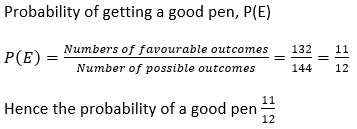5. If the probability of winning a game is 5/11, what is the probability of losing ?
Solution:-
we have given that
Given probability of winning the game, P(E) = 5/11
As We know that,6. Two players, Sania and Sonali play a tennis match. It is known that the probability of Sania winning the match is 0.69. What is the probability of Sonali winning ?
Solution:-
We have given that
Probability of Sania winning the match, P(E) = 0.69
Therefore , Probability of Sonali winning = Probability of Sania losing,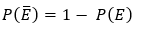Then the probability of Sonali winning = 1-0.69
Therefore , the probability of Sonali winning = 0.31

7. A bag contains 3 red balls and 5 black balls. A ball is drawn at random from a bag. What is the probability that the ball drawn is .
(i) red ball ?
(ii) not red ball ?
Solution:-
(i) We have given that
Number of red balls = 3
Number of black balls = 5
Total number of balls = 3+5 = 8
Probability that the ball drawn is red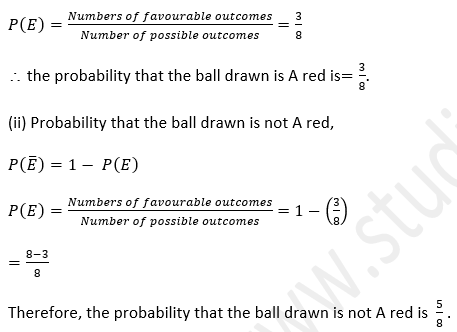8. There are 40 students in Class X of a school of which 25 are girls and the others are boys. The class teacher has to select one student as a class representative. She writes the name of each student on a separate card, the cards being identical. Then she puts cards in a bag and stirs them thoroughly. She then draws one card from the bag. What is the probability that the name written on the card is the name of
(i) a girl ?
(ii) a boy ?
Solution:-
We have given that,
Total number of students = 40
Number of girls = 25
Number of boys = 40-25 = 15
Now,
therefore,(i) Probability of getting a girl,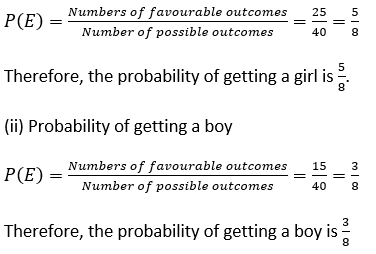9. A letter is chosen from the word ‘TRIANGLE’. What is the probability that it is a vowel ?
Solution:-
Total Number of vowels in the word ‘TRIANGLE’ = 3
Total number of letters = 8
We have given that,
Probability that the letter chosen is a vowel ,10. A letter of English alphabet is chosen at random. Determine the probability that the letter is a consonant
Solution:-
We have given that,
Total number of alphabets = 26
Number of vowels = 5
Then,
Total number of consonants = 26-5 = 21
Now, Probability that the letter chosen is a consonant ,11. A bag contains 5 black ball , 7 red ball  and 3 white balls. A ball is drawn at random from the bag, find the probability that the ball drawn is:
(i) red
(ii) black or white
(iii) not black.
Solution:-
We have given that ,
Total Number of black balls = 5
Total Number of red balls = 7
Total Number of white balls = 3
Total number of balls = 5+7+3 = 15
(i) Probability that the ball drawn is red,12. A box contains 7 blue, 8 white and 5 black marbles. If a marble is drawn at random from the box, what is the probability that it will be
(i) black?
(ii) blue or black?
(iii) not black?
(iv) green?
Solution:-
We have given that,
Number of blue marbles = 7
Number of white marbles = 8
Number of black marbles = 5
Total number of marbles = 7+8+5 = 20
(i) Probability of getting black ,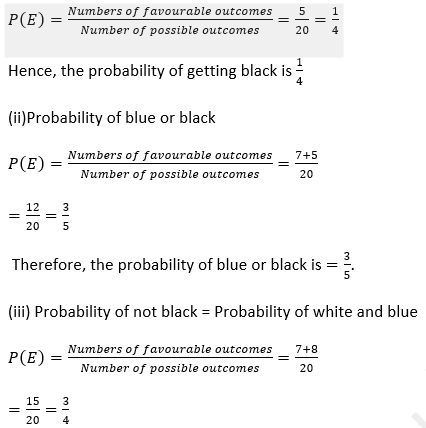Therefore,  the probability of not black is =3/4.
(iv) Since there are no green marbles in the box, the probability of green is 0.

13. A bag contains 6 red balls, 8 white balls, 5 green balls and 3 black balls. One ball is drawn at random from the bag. Find the probability that the ball is :
(i) white ball
(ii) red or black ball
(iii) not green ball
(iv) neither white nor black ball
Solution:-
We have given that,
Total Number of red balls = 6
Total Number of white balls = 8
Total Number of green balls = 5
Total Number of black balls = 3
Then the Total number of marbles = 6+8+5+3 = 22
(i)Probability of white balls,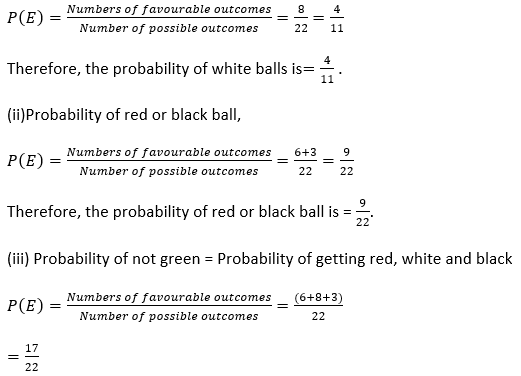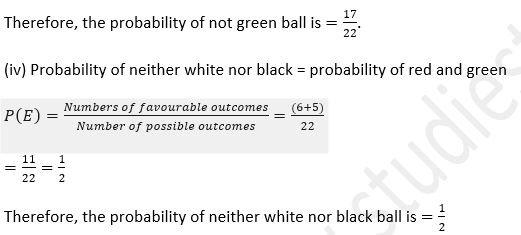14. A piggy bank contains hundred 50 p coins, fifty Rs 1 coins, twenty Rs 2 coins and ten Rs 5 coins. It is equally likely that one of the coins will fall down when the bank is turned upside down, what is the probability that the coin
(i) will be a 50 p coin?
(ii) will not be Rs 5 coin?
Solution:-
As we have given that,
Total Number of 50 paisa coins = 100
Total Number of 1 rupee coins = 50
Total Number of 2 rupee coins = 20
Total Number of 5 rupee coins = 10
Then, the Total number of coins = 100+50+20+10 = 180
(i) Probability of getting a 50 paisa coin,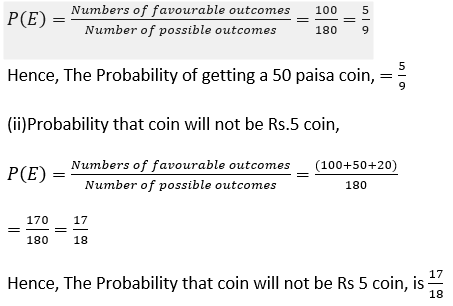15. A carton consists of 100 shirts of which 88 are good, 8 have minor defects and 4 have major defects. Peter, a trader, will only accept the shirts which are good, but Salim, another trader, will only reject the shirts which have major defects. One shirts is drawn at random from the carton. What is the probability that
(i) it is acceptable to Peter ?
(ii) it is acceptable to Salim ?
Solution:
As we have given that,
Total number of shirts = 100
Number of good shirts = 88
Number of shirts with minor defects = 8
Number of shirts with major defects = 4
Peter accepts only good shirts.
So the number of shirts he accepts = 88
Salim will reject shirts with only major defects. So number of shirts he accepts = 88+8 = 96
(i) Probability that it is acceptable to Peter,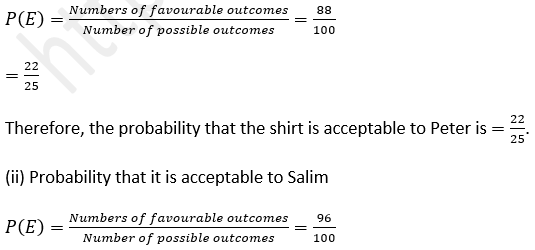= 24/25
Therefore, the probability that the shirt is acceptable to Salim is=24/25

16. A die is thrown once. What is the probability that the
(i) number is even
(ii) number is greater than 2 ?
Solution:-
As we have given that,
When a die is thrown, the possible outcomes are 1,2,3,4,5,6.
So the Sample space = { 1,2,3,4,5,6}
therefore, Number of possible outcomes = 6
Even numbers are (2,4,6). Number of favourable outcomes = 3
(i)Probability that the number is even,= 1/2
Therefore, The probability of even number when a die thrown once is =1/2.
(ii)When the number is greater than 2,
the possible outcomes are 3,4,5,6
Number of favourable outcomes = 4
Therefore, Probability that the number is greater than 2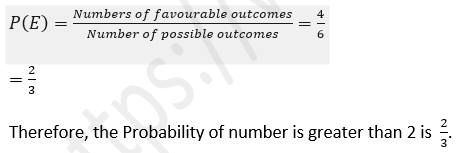17. In a single throw of a die, find the probability of getting:
(i) an odd number
(ii) a number less than 5
(iii) a number greater than 5
(iv) a prime number
(v) a number less than 8
(vi) a number divisible by 3
(vii) a number between 3 and 6
(viii) a number divisible by 2 or 3.
Solution:-
As we have given that,
When a die is thrown, the possible outcomes are 1,2,3,4,5,6.
Therefore, Number of possible outcomes = 6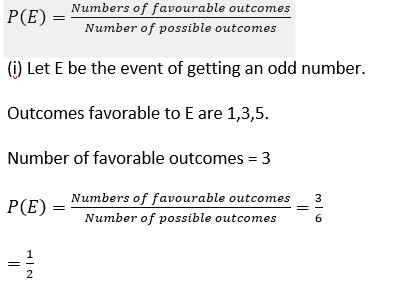Therefore, the probability of getting an odd number is  1/2  .
(ii)Let E be event of getting a number less than 5.
Outcomes favourable to E are 1,2,3,4
Number of favourable outcomes = 4(iv) Let E be the event of getting a prime number .
Outcomes favourable to E is 2,3,5.
Number of favourable outcomes = 3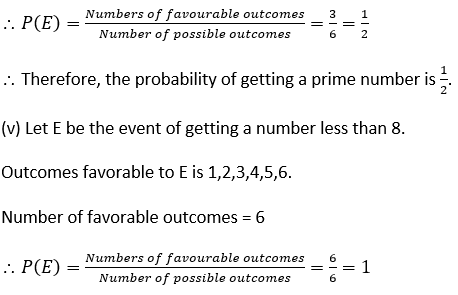Therefore, the probability of getting a number less than 8 is 1.
(vi) Let E be the event of getting a number divisible by 3.
Outcomes favourable to E is 3,6.
Number of favourable outcomes = 2(viii) Let E be the event of getting a number divisible by 2 or 3.
Outcomes favourable to E is 2,3,4,6.
Number of favourable outcomes = 4(viii) Let E be the event of getting a number divisible by 2 or 3.
Outcomes favourable to E is 2,3,4,6.
Number of favourable outcomes = 4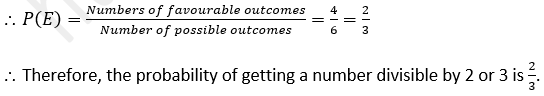18. A die has 6 faces marked by the given numbers as shown below: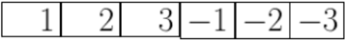The die is thrown once. What is the probability of getting
(i) a positive integer.
(ii) an integer greater than – 3.
(iii) the smallest integer ?
Solution:-
As we have given that,
When a die is thrown, the possible outcomes are {1,2,3,-1,-2,-3}
So, the Number of possible outcomes = 6
(i) Let E be the event of getting a positive integer.
Outcomes favourable to E are {1,2,3}
Number of favourable outcomes = 3(iii) Let E be the event of getting the smallest integer.
Outcomes favorable to E is -3.
Number of favorable outcomes = 1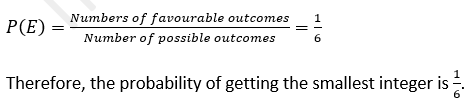19. A game of chance consists of spinning an arrow which comes to rest pointing at one of the numbers 1, 2, 3, 4, 5, 6, 7, 8 (shown in the adjoining figure) and these are equally likely outcomes. What is the probability that it will point at
(i) 8 ?
(ii) an odd number ?
(iii) a number greater than 2?
(iv) a number less than 9?Solution:-
As we have given that,
The possible outcomes of the game are {1,2,3,4,5,6,7,8}
Number of possible outcomes = 8
(i) Let E be the event of arrow pointing 8.
Outcomes favourable to E is 8.
Number of favourable outcomes = 1
Therefore,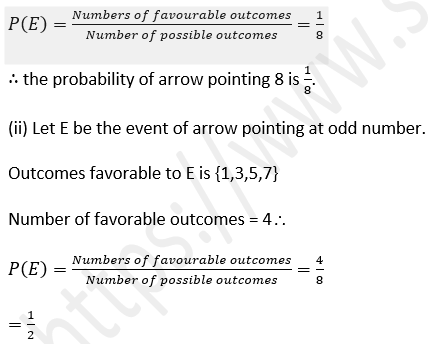Therefore, the probability of arrow pointing at odd number is   1/2
(iii) Let E be the event of arrow pointing a number greater than 2.
Outcomes favourable to E are {3,4,5,6,7,8}
Number of favourable outcomes = 620. Find the probability that the month of January may have 5 Mondays in
(i) a leap year
(ii) a non-leap year.
Solution:-
As we have given that,
Number of days in Leap year is = 366 days.
Number of days in January = 31
Total number of January month types = 7
Number of January months with 5 Mondays = 3
(i) Probability that the month of January have 5 Mondays in leap year = 3/7
(ii) Probability that the month of January have 5 Mondays in a non leap year =3/7
Hence, the probability of month of January have 5 Mondays has 3/7 and a non-leap year has also 3/7 .

21. Find the probability that the month of February may have 5 Wednesdays in
(i) a leap year
(ii) a non-leap year.
Solution:-
As We have given that,
There are 7 ways in which the month of February can occur, each starting with a different day of the week.
(i) Only 1 way is possible for 5 Wednesdays to occur in February with 29 days.
i.e, month starts on Wednesday.
Therefore, Probability that February have 5 Wednesdays in a leap year =1/7
(ii) In a non leap year, it is not possible to have 5 Wednesdays for February.
Therefore, the probability that February have 5 Wednesdays in a non leap year = 0.

22. Sixteen cards are labeled as a, b, c,…, m, n, o, p. They are put in a box and shuffled. A boy is asked to draw a card from the box. What is the probability that the card drawn is:
(i) a vowel
(ii) a consonant
(iii) none of the letters of the word median.
Solution:-
As we have given that,
The possible outcomes are {a,b,c,d,e,f,g,h,i,j,k,l,m,n,o,p}
Number of possible outcomes = 16
(i) Let E be the event of getting a vowel.
Outcomes favourable to E are { a,e,i,o}
Number of favourable outcomes = 4(iii) Let E be the event of getting none of the letters of word median.
Outcomes favourable to E are {b,c,f,g,h,j,k,l,o,p}
Number of favourable outcomes = 1023. An integer is chosen between 0 and 100. What is the probability that it is
(i) divisible by 7?
(ii) not divisible by 7?
Solution:-
As we have given that,
Number of integers between 0 and 100 = 99
Number of possible outcomes = 99
(i) Let E be the event of getting an integer divisible by 7.
Outcomes favourable to E are {7,14,21,28,35,42,49,56,63,70,77,84,91,98}
Number of favourable outcomes = 14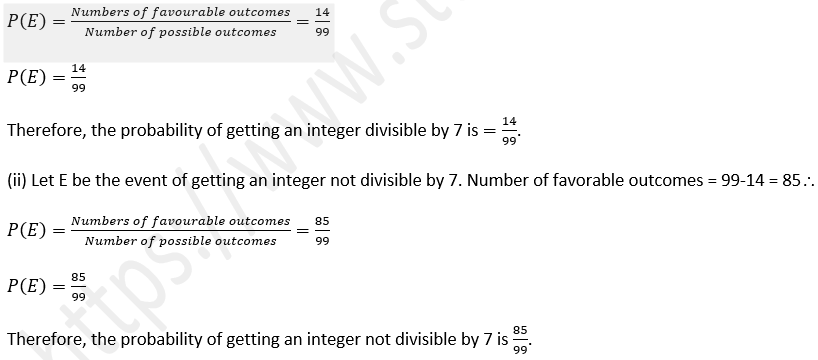24. Cards marked with numbers 1, 2, 3, 4,…20 are well shuffled and a card is drawn at random. What is the probability that the number on the card is
(i) a prime number
(ii) divisible by 3
(iii) a perfect square ?
Solution:-
As we have given that,
The possible outcomes are {1,2,3,….20}
Number of possible outcomes = 20
(i) Let E be the event of getting the number on the card is a prime number.
Outcomes favourable to E are {2,3,5,7,11,13,17,19}
Number of favourable outcomes = 8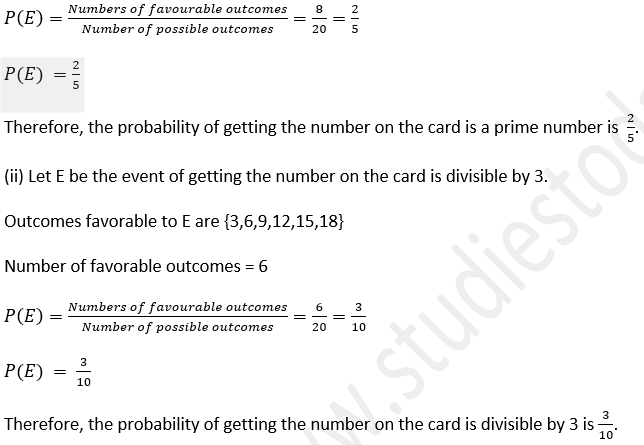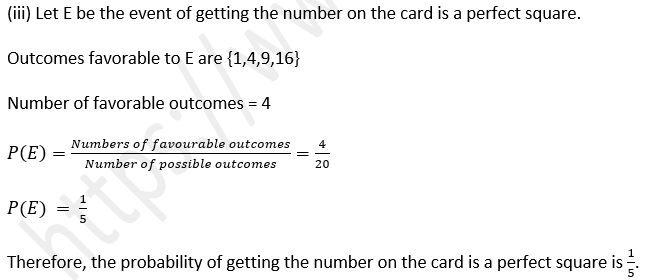25. A box contains 25 cards numbered 1 to 25. A card is drawn from the box at random. Find the probability that the number on the card is
(i) even
(ii) prime
(iii) multiple of 6.
Solution:-
We have given that,
The possible outcomes are {1,2,3,4 ….25}
Number of possible outcomes = 25
(i) Let E be the event of getting the number on the card is an even number.
Outcomes favourable to E are {2,4,6,8,10,12,14,16,18,20,22,24}
Number of favourable outcomes = 12Therefore, the probability of getting the number on the card is a prime number is =9/25.
(iii) Let E be the event of getting the number on the card is a multiple of 6.
Outcomes favourable to E are {6,12,18,24}
Number of favourable outcomes = 4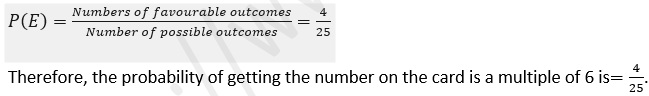26. A box contains 15 cards numbered 1, 2, 3,…..15 which are mixed thoroughly. A card is drawn from the box at random. Find the probability that the number on the card is :
(i) Odd
(ii) prime
(iii) divisible by 3
(iv) divisible by 3 and 2 both
(v) divisible by 3 or 2
(vi) a perfect square number.
Solution:-
As we have given that,
The possible outcomes are {1,2,3,4…15}
Number of possible outcomes = 15
(i) Let E be the event of getting the number on the card is odd.
Outcomes favourable to E are {1,3,5,7,9,11,13,15}
Number of favourable outcomes = 8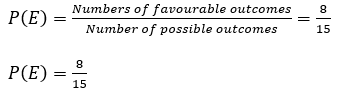Therefore, the probability of getting the number on the card is an odd number is 8/15.
(ii) Let E be the event of getting the number on the card is prime.
Outcomes favorable to E are {2,3,5,7,11,13}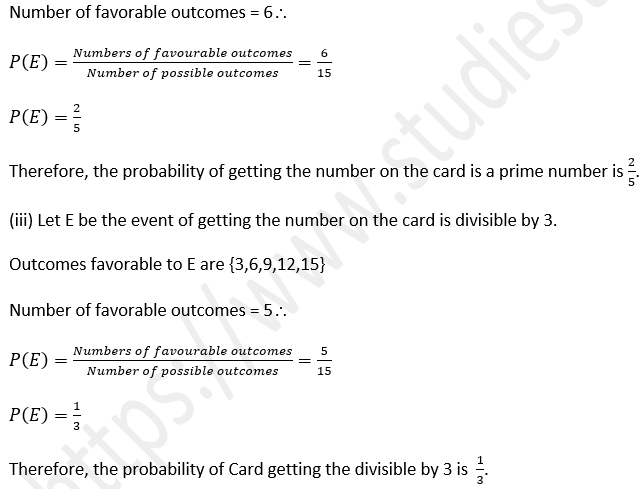(iv) Let E be the event of getting the number on the card is divisible by 3 and 2 both.
Outcomes favourable to E are {6,12}
Number of favourable outcomes = 2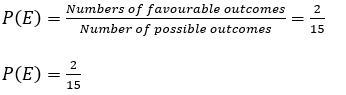Therefore, the probability of Card getting the number is divisible by 3 and 2 both is 2/15
(v) Let E be the event of getting the number on the card is divisible by 3 or 2
Outcomes favourable to E are {2,3,4,6,8,9,10,12,14,15}
Number of favourable outcomes = 10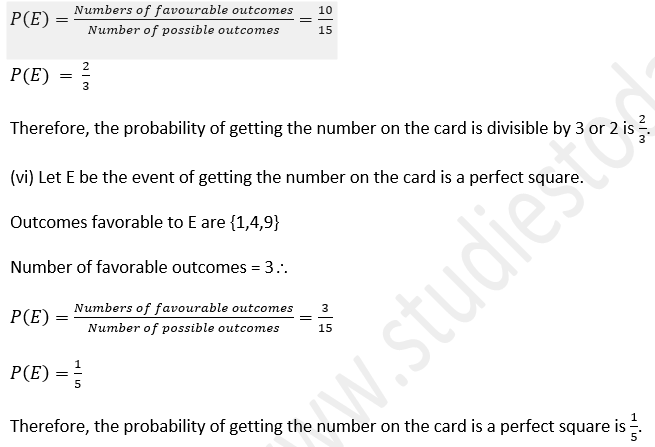27. A box contains 19 balls bearing numbers 1, 2, 3,…., 19. A ball is drawn at random from the box. Find the probability that the number on the ball is :
(i) a prime number
(ii) divisible by 3 or 5
(iii) neither divisible by 5 nor by 10 (iv) an even number.
Solution:-
As we have given that,
The possible outcomes are {1,2,3,4…19}
Number of possible outcomes = 19
(i) Let E be the event of getting the number on the ball is a prime number.
Outcomes favourable to E are {2,3,5,7,11,13,17,19}
Number of favourable outcomes = 8Therefore, the probability of getting the number on the card is divisible by 3 or 5 is=819.
(iii) Let E be the event of getting the number on the ball is neither divisible by 5 nor by 10.
Outcomes favorable to E are {1,2,3,4,6,7,8,9,11,12,13,14,16,17,18,19}P(E)=9/19
Therefore, the probability of getting the number on the card is an even number is 9/19

28. Cards marked with numbers 13, 14, 15, …, 60 are placed in a box and mixed thoroughly. One card is drawn at random from the box. Find the probability that the number on the card drawn is
(i) divisible by 5
(ii) a perfect square number.
Solution:-
As we have given that,
The possible outcomes are {13,14,15,…60}
Number of possible outcomes = 48
(i) Let E be the event of getting the number on the card is divisible by 5.
Outcomes favourable to E are {15,20,25,30,35,40,45,50,55,60}
Number of favourable outcomes = 10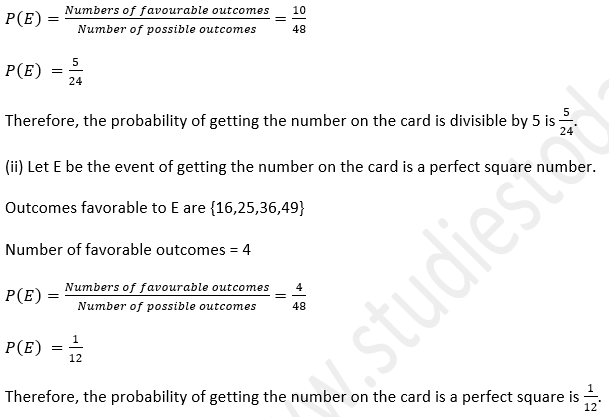29. Tickets numbered 3, 5, 7, 9,…., 29 are placed in a box and mixed thoroughly. One ticket is drawn at random from the box. Find the probability that the number on the ticket is
(i) a prime number
(ii) a number less than 16
(iii) a number divisible by 3.
Solution:-
As we have given that,
The possible outcomes are {3,5,7,9..…29}
Number of possible outcomes = 14
(i) Let E be the event of getting the number on the ticket is a prime number.
Outcomes favourable to E are {3,5,7,11, ,13,17,19,23,29}
Number of favourable outcomes = 9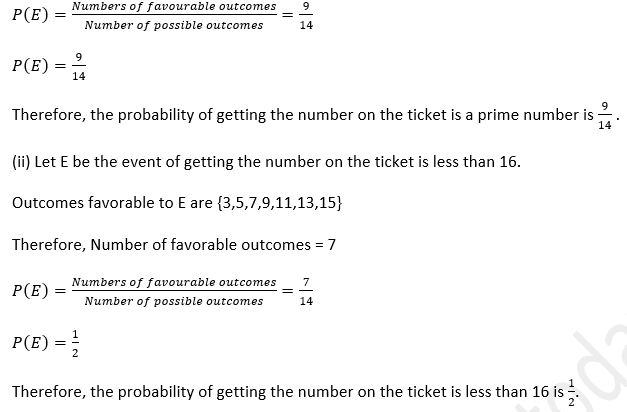(iii) Let E be the event of getting the number on the ticket is a number divisible by 3.
Outcomes favorable to E are {3,9,15,21,27}
Number of favorable outcomes = 530. A box contains 90 discs which are numbered from 1 to 90. If one disc is drawn at random from the box, find the probability that it bears
(i) a two-digit number
(ii) a perfect square number
(iii) a number divisible by 5.
Solution:-
As  we have given that,
The possible outcomes are {1,2,3,…90}
Number of possible outcomes = 90
(i) Let E be the event of getting the number on the disc is a two-digit number.
Outcomes favourable to E are {10,11,12,….90}
Number of favourable outcomes = 81(iii) Let E be the event of getting the number on the disc is a number divisible by 5.
Outcomes favourable to E are {5,10,15,20,25,30,35,40,45,50,55,60,65,70,75,80,85,90}
Therefore ,Number of favourable outcomes = 18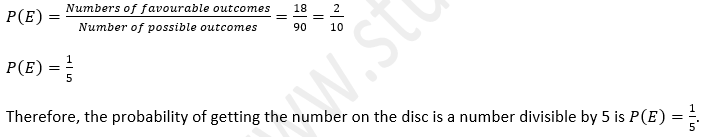31. Cards marked with numbers 2 to 101 are placed in a box and mixed thoroughly. One card is drawn at random from this box. Find the probability that the number on the card is
(i) an even number
(ii) a number less than 14
(iii) a number which is a perfect square
(iv) a prime number less than 30.
Solution:-
As  we have given that,
The possible outcomes are {2,3,…101}
Number of possible outcomes = 100
(i) Let E be the event of getting the number on the card is an even number.
Outcomes favourable to E are {2,4,6,8…100}
Number of favourable outcomes = 50(iii) Let E be the event of getting the number on the card is a perfect square.
Outcomes favourable to E are {4,9,16,25,36,49,64,81,100}
Number of favourable outcomes = 9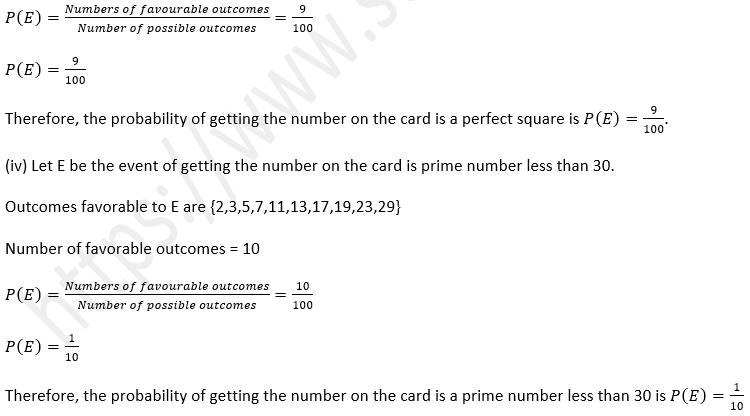32. A bag contains 15 balls of which some are white and others are red. If the probability of drawing a red ball is twice that of a white ball, find the number of white balls in the bag.
Solution:-
As we have given that,
Total number of balls in the bag = 15.
Let the number of white balls be x.
Then number of red balls = 15-x.
The probability of drawing a white ball =x/15.33. A bag contains 6 red balls and some blue balls. If the probability of drawing a blue ball is twice that of a red ball, find the number of balls in the bag.
Solution:-
As we have given that
Number of red balls = 6
Let number of blue balls be x.
Total number of balls = 6+x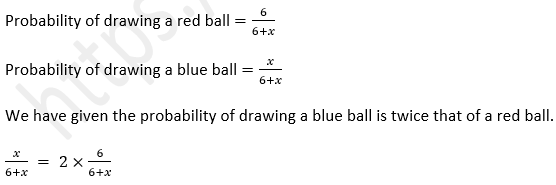x = 12.
Then the total number of balls = x+6 = 12+6 = 18.
Therefore, the total number of balls in the bag is 18.

34. A bag contains 24 balls of which x are red, 2x are white and 3x are blue. A ball is selected at random. Find the probability that it is
(i) white
(ii) not red.
Solution:-
As we have given that,
Total number of balls = 24
Number of red balls = x.
Number of white balls = 2x.
Number of blue balls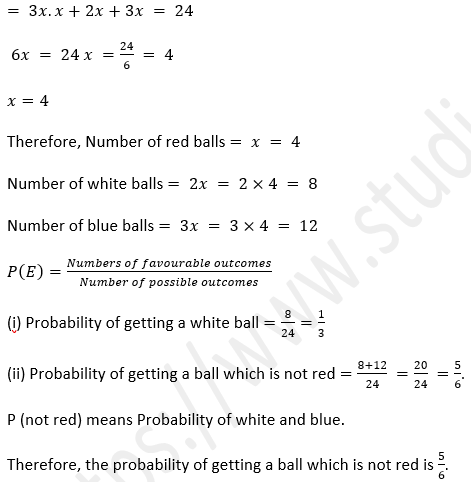35. A card is drawn from a well-shuffled pack of 52 cards. Find the probability of getting:
(ii) a jack .
(iii) a king of red colour
(iv) a card of diamond
(v) a king or a queen
(vi) a non-face card
(vii) a black face card
(viii) a black card
(ix) a non-ace
(x) non-face card of black colour
(xi) neither a spade nor a jack
(xii) neither a heart nor a red king
Solution:-
As we have given that,
Total number of cards = 52.
So number of possible outcomes = 52.
(i) Let E be the event of getting ‘2’ of spades.
As we know that,
There will be only one card of ‘2’ spades.
Number of favourable outcomes = 1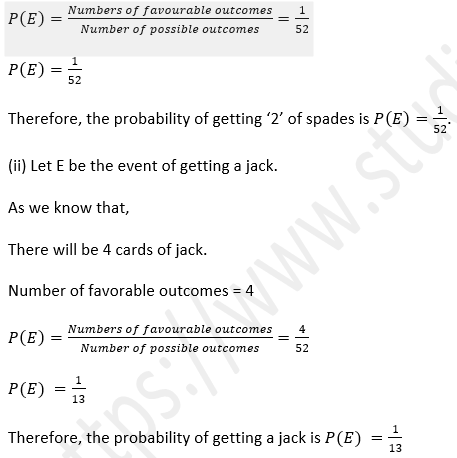(iii) Let E be the event of getting a king of red color.
As we know that,
There will be 2 cards of king of red color.
Number of favorable outcomes = 2(v) Let E be the event of getting a king or a queen.
As we know that,
There will be 4 cards of king and 4 cards of queen.
Number of favorable outcomes = 4+4 = 8(vii) Let E be the event of getting a black face card.
As we know that,
There will be 6 black face cards.
Number of favorable outcomes = 6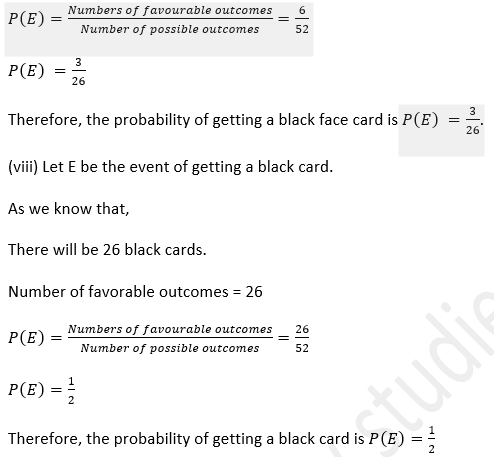(ix) Let E be the event of getting a non-ace card.
As we know that,
There will be 48 non-ace cards.
Number of favorable outcomes = 48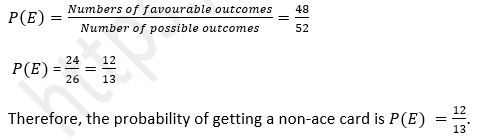(x) Let E be the event of getting a non-face card of black colour.
As we know that,
There will be 20 non face cards.
Number of favorable outcomes = 20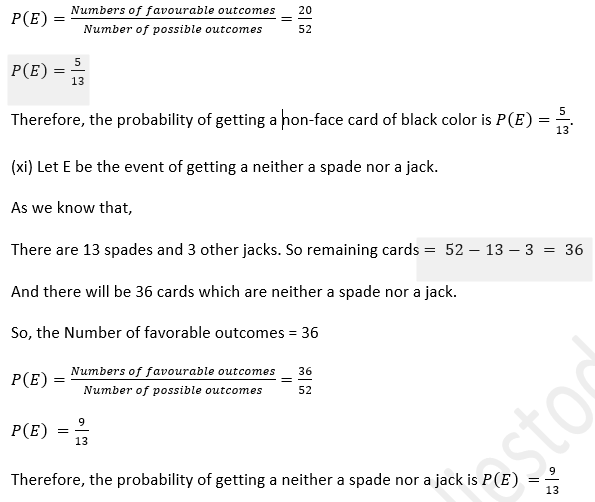(xii) Let E be the event of getting a neither a heart nor a red king.
As we know that,
There are 13 heart cards and 1 other red king.
So remaining cards = 52−13−1 = 38
There will be 38 cards which are neither a heart nor a red king.
Number of favorable outcomes = 38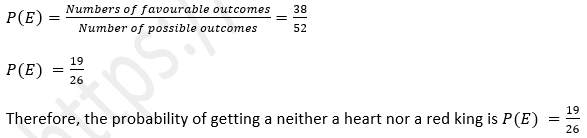36. All the three face cards of spades are removed from a well-shuffled pack of 52 cards. A card is then drawn at random from the remaining pack. Find the probability of getting
(i) a black face card
(ii) a queen
(iii) a black card
(iv) a heart
(vi) ‘9’ of black colour.
Solution:-
As we have given that
Total number of cards = 52-3 = 49.         [since 3 face cards of spade are removed]
So number of possible outcomes = 49.
(i) Let E be the event of getting black face card.
As we know that,
There will be 3 black face cards of clubs .
So, the Number of favorable outcomes = 3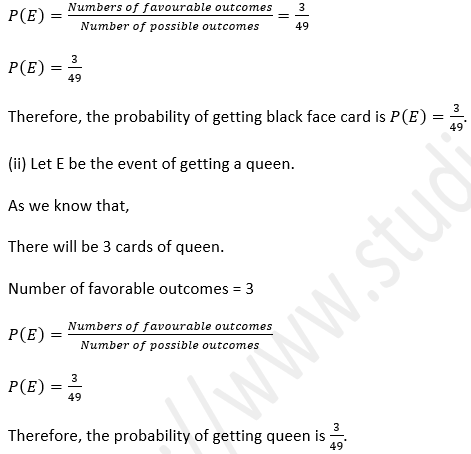(iii) Let E be the event of getting a black card.
As we know that,
There will be 23 black cards remaining since 3 spades are removed.
Number of favourable outcomes = 23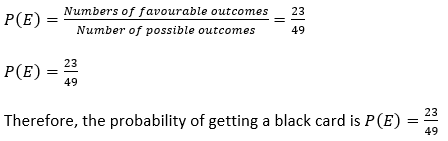(iv) Let E be the event of getting a heart.
As we know that,
There will be 13 hearts.
Number of favourable outcomes = 13(vi) Let E be the event of getting ‘09’ of black colour.
As we know that,
There will be 2 such cards.
Number of favourable outcomes = 237. From a pack of 52 cards, a blackjack, a red queen and two black kings fell down. A card was then drawn from the remaining pack at random. Find the probability that the card drawn is
(i) a black card
(ii) a king
(iii) a red queen.
Solution:
As we have given that
Total number of cards = 52-4 = 48     [∵4 cards fell down]
So number of possible outcomes = 48
(i) Let E be the event of getting black card.
As we know that,
There will be 23 black cards since a black jack and 2 black kings fell down.
Number of favourable outcomes = 23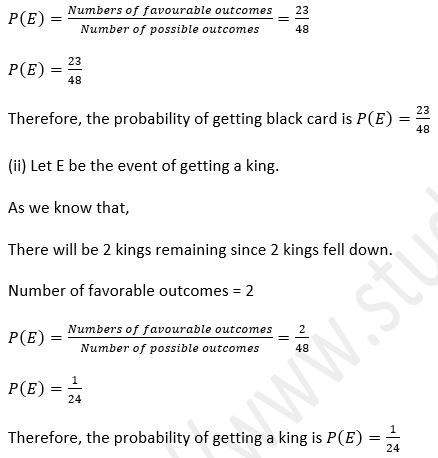(iii) Let E be the event of getting red queen.
As we know that,
There will be 1 red queen.
Number of favorable outcomes = 1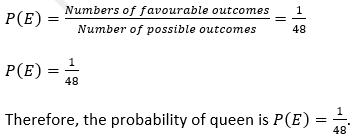38. Two coins are tossed once. Find the probability of getting:
(ii) at least one tail.
Solution:-
As we know that,
When 2 coins are tossed,
The possible outcomes are HH. HT, TH, TT.
So, the Number of possible outcomes = 4
(i)Let E be an event of getting 2 heads.
Favourable outcomes = HH
Number of favourable outcomes = 139. Two different coins are tossed simultaneously. Find the probability of getting :
(i) two tails
(ii) one tail
(iii) no tail
(iv) at most one tail.
Solution:-
As we know that,
When 2 coins are tossed, the possible outcomes are HH. HT, TH, TT.
So the Number of possible outcomes = 4
(i) Let E be an event of getting 2 tails.
Favourable outcomes = TT
So, the Number of favourable outcomes = 1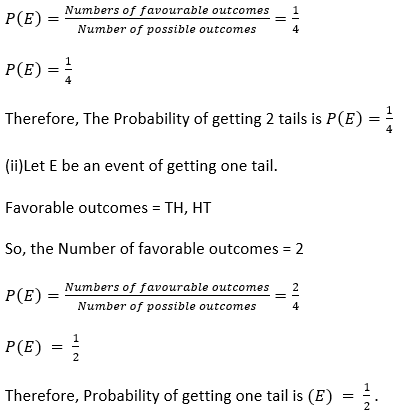(iii)Let E be an event of getting no tails.
Favourable outcomes = HH
So, the Number of favourable outcomes = 140. Two different dice are thrown simultaneously. Find the probability of getting:
(i) a number greater than 3 on each dice
(ii) an odd number on both dice.
Solution:-
As we know that,
When two dice are thrown simultaneously, the sample space of the experiment is
{(1,1),(1,2),(1,3),(1,4),(1,5),(1,6),(2,1),(2,2),(2,3),(2,4),(2,5),(2,6),(3,1),(3,2),(3,3),(3,4),(3,5),  (3,6),(4,1),(4,2),(4,3),(4,4),(4,5),(4,6),(5,1),(5,2),(5,3),(5,4),(5,5),(5,6),(6,1),(6,2),(6,3),(6,4),(6,5),(6,6)}
So there are 36 equally likely outcomes. Possible number of outcomes = 36.
(i)Let E be an event of getting a number greater than 3 on each dice.
Favourable outcomes = {(4,4),(4,5),(4,6),(5,4),(5,5),(5,6),(6,4),(6,5),(6,6)}
So, the Number of favourable outcomes = 941. Two different dice are thrown at the same time. Find the probability of getting :
(i) a doublet
(ii) a sum of 8
(iii) sum divisible by 5
(iv) sum of at least 11.
Solution-
As we have given that,
When two dice are thrown simultaneously, the sample space of the experiment is
{(1,1),(1,2),(1,3),(1,4),(1,5),(1,6),(2,1),(2,2),(2,3),(2,4),(2,5),(2,6),(3,1),(3,2),(3,3),(3,4),(3,5),(3,6),(4,1),(4,2),(4,3),(4,4),(4,5),(4,6),(5,1),(5,2),(5,3),(5,4),(5,5),(5,6),(6,1),(6,2),(6,3),(6,4),(6,5),(6,6)} So there are 36 equally likely outcomes.
The Possible number of outcomes = 36.
(i)Let E be an event of getting a doublet.
Favourable outcomes = {(1,1), (2,2),(3,3), (4,4), (5,5),(6,6)}
So, the number of favourable outcomes = 6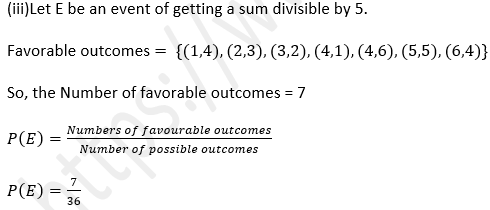Therefore, Probability of getting a sum divisible by 5 is P(E) =736.
(iv) Let E be an event of getting a sum of at least 11.
Favourable outcomes = {(5,6),(6,5), (6,6)}
So, the Number of favourable outcomes = 3